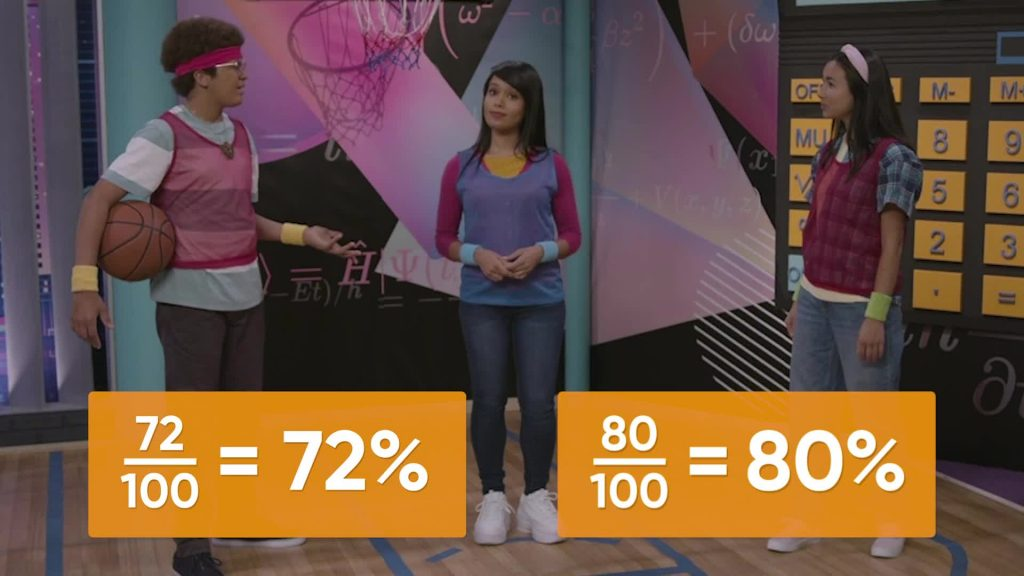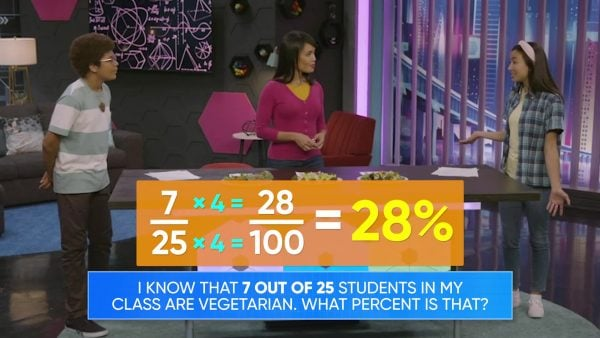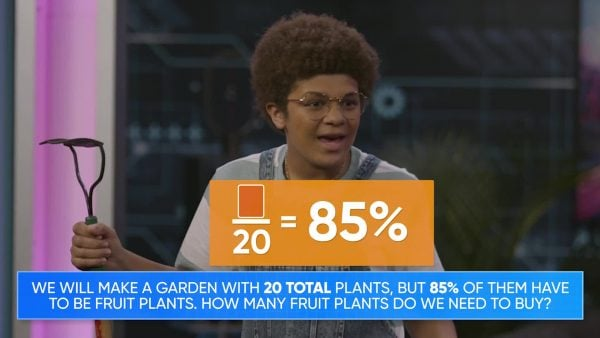Percents | Math for Grades 6, 7, & 8
1%
It was processed successfully!WHAT ARE PERCENTS?

Percents are special ratios where the whole is always 100. You use the % symbol to represent “percent.” 5 out of 100 or 5 : 100 is 5%.

To better understand percents…

WHAT ARE PERCENTS?. Percents are special ratios where the whole is always 100. You use the % symbol to represent “percent.” 5 out of 100 or 5 : 100 is 5%. To better understand percents…

## LET’S BREAK IT DOWN!Amari and Emily are shooting hoops. Amari scored 72 out of 100 shots. Emily scored 80 out of 100 shots. How can you represent these as ratios and percents? A ratio is a relationship between two numbers. Ratios can be part-part or part-whole. Because we are looking at the number of scores (part) out of the number of shots (whole), we know that the ratio is part-whole. This is important because percents can only represent part-whole ratios. We can represent Amaris score as 72 :100 or [ggfrac]72/100[/ggfrac] or [ggfrac]72/100[/ggfrac] or 72%. We can also represent Amari's score as a decimal number where 1 is the whole, as 72 hundredths or 0.72. [b]Try this yourself: What are all the ways you can represent Emily's score? What is her score as a percent and as a decimal?[/b]

Playing Basketball Amari and Emily are shooting hoops. Amari scored 72 out of 100 shots. Emily scored 80 out of 100 shots. How can you represent these as ratios and percents? A ratio is a relationship between two numbers. Ratios can be part-part or part-whole. Because we are looking at the number of scores (part) out of the number of shots (whole), we know that the ratio is part-whole. This is important because percents can only represent part-whole ratios. We can represent Amaris score as 72 :100 or [ggfrac]72/100[/ggfrac] or [ggfrac]72/100[/ggfrac] or 72%. We can also represent Amari's score as a decimal number where 1 is the whole, as 72 hundredths or 0.72. [b]Try this yourself: What are all the ways you can represent Emily's score? What is her score as a percent and as a decimal?[/b]

### Class LunchWe are going on a class trip to the local science museum and need to plan for lunch. We surveyed the food preferences of the class, and 5 out of 20 students said they are vegetarian. We can represent this ratio as 5 :20 or [ggfrac]5/20[/ggfrac] or [ggfrac]5/20[/ggfrac]. But how do we represent this ratio as a percent? First we need to convert our ratio to an equivalent ratio out of 100. To make an equivalent ratio we multiply both parts of the ratio by the same number. We know that 20×5=100, so we need to multiply the numerator by 5 as well: 5×5=25. That means the equivalent ratio is 25 :100 or [ggfrac]25/100[ggfrac] or [ggfrac]25/100[/ggfrac] or 25%. So 25% of the students in the class are vegetarians. [b]Try this yourself: 7 out of 25 students in Emily's class are vegetarian. What percent of her class is vegetarian?[/b]

Class Lunch We are going on a class trip to the local science museum and need to plan for lunch. We surveyed the food preferences of the class, and 5 out of 20 students said they are vegetarian. We can represent this ratio as 5 :20 or [ggfrac]5/20[/ggfrac] or [ggfrac]5/20[/ggfrac]. But how do we represent this ratio as a percent? First we need to convert our ratio to an equivalent ratio out of 100. To make an equivalent ratio we multiply both parts of the ratio by the same number. We know that 20×5=100, so we need to multiply the numerator by 5 as well: 5×5=25. That means the equivalent ratio is 25 :100 or [ggfrac]25/100[ggfrac] or [ggfrac]25/100[/ggfrac] or 25%. So 25% of the students in the class are vegetarians. [b]Try this yourself: 7 out of 25 students in Emily's class are vegetarian. What percent of her class is vegetarian?[/b]

### Calculating Homework### Planting a GardenWe can also find the amount of a part for a given percent. We need to plant a garden with 20 plants in total, and 85% of the plants need to be fruit plants. We start by setting up an equation with the information we already know: [ggfrac] number of fruit plants/ total number of plants[/ggfrac]=[ggfrac]percent/100[/ggfrac]. Then we fill in the values we know, to get [ggfrac]n/20[/ggfrac]=[ggfrac]85/100[/ggfrac], where [i]n[/i] represents the number of fruit plants. We can solve this problem by multiplying both the numerator and denominator by the same number. We can see that 20×5=100, so [i]n[/i]×5=85. Now we need to find the value of [i]n[/n]. We know that 17×5=85, so [i]n[/i] must equal 17. We need to have 17 fruit plants in the garden. [b]Try this yourself: If you are planting a garden with 25 plants, and 72% need to be vegetables, how many vegetable plants do you need?[/b]

Planting a Garden We can also find the amount of a part for a given percent. We need to plant a garden with 20 plants in total, and 85% of the plants need to be fruit plants. We start by setting up an equation with the information we already know: [ggfrac] number of fruit plants/ total number of plants[/ggfrac]=[ggfrac]percent/100[/ggfrac]. Then we fill in the values we know, to get [ggfrac]n/20[/ggfrac]=[ggfrac]85/100[/ggfrac], where [i]n[/i] represents the number of fruit plants. We can solve this problem by multiplying both the numerator and denominator by the same number. We can see that 20×5=100, so [i]n[/i]×5=85. Now we need to find the value of [i]n[/n]. We know that 17×5=85, so [i]n[/i] must equal 17. We need to have 17 fruit plants in the garden. [b]Try this yourself: If you are planting a garden with 25 plants, and 72% need to be vegetables, how many vegetable plants do you need?[/b]

## PERCENTS VOCABULARY

Ratio
A multiplicative comparison of two amounts.
Multiplicative
Related to or based on multiplication.
Part-part ratio
A ratio that compares the size of two parts from the same whole.
Part-whole ratio
A ratio that compares the size of one part to the size of the whole. Fractions are a form of part-whole ratio.
Numerator
In a part-whole ratio or in a fraction, the numerator represents the number of parts.
Denominator
In a part-whole ratio or in a fraction, the denominator represents the number of parts the whole is partitioned into.
Ratios that are multiples of one another. Multiplying both the numerator and denominator of the ratio by the same value produces an equivalent ratio.
A part-whole ratio out of 100.

## PERCENTS DISCUSSION QUESTIONS

### What is a percent?

A percent is a special part-whole ratio where the whole is 100.

### What is 60% of 100? How do you know?

Percent means per 100, so 60 percent means 60 per 100. So 60% of 100 is 60.

### What is 60% of 50? How do you know?

I can answer this by reasoning about equivalent ratios. I know that 60% of 100 is 60. I also know that half of 100 is 50. To keep the ratios equivalent I need to multiply or divide both the numerator and denominator of my ratio by the same value. Since 50 is half of 100, and 30 is half of 60, 60% of 50 must be 30.

### What is 28% as a decimal?

I know that 28% means I have 28 out of 100. This also means I have 28 hundredths. I write 28 hundredths as 0.28, so 0.28 is the decimal value of 28%.

### You have stacked 20 logs so far. You are 80% of the way through stacking the wood pile. How many logs are in the whole pile?

First I need to set up an equation [ggfrac]20/n[/ggfrac]=[ggfrac]80/100[/ggfrac] and then use proportional reasoning. I know that 20×4=80, and to keep my ratios equivalent, I need to multiply the numerator and denominator by the same value. This means [i[]n[/i]×4=100, so since 25×4=100 there must be 25 logs in the whole pile.
X

## Success

We’ve sent you an email with instructions how to reset your password.
Ok